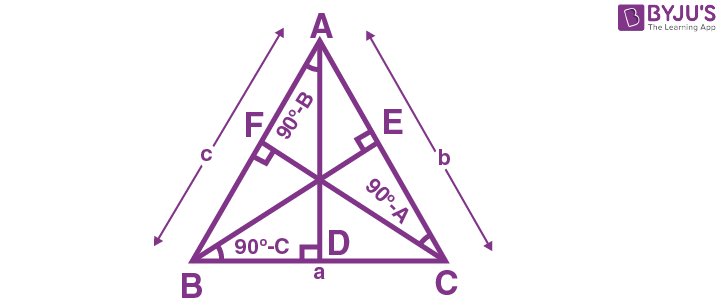Checkout JEE MAINS 2022 Question Paper Analysis : Checkout JEE MAINS 2022 Question Paper Analysis :

# If in a ∆ABC, the altitudes from the vertices A, B, C on the opposite side are in HP, then sin A, sin B, and sin C are in

1) HP

2) Arithmetico – Geometric Progression

3) AP

4) GP

Solution:

Consider ∆BDA,

cos (90 – B) = AD/cSimilarly, BE = a sin C

CF = b sinA

Given AD, BE, CF are in HP.

So c sin B, a sin C, b sin A are in HP.

=> 1/sin C sin B, 1/sin A sin C, 1/sin B sin A are in AP.

Multiply by sin A sin B sin C

=> sin A, sin B sin C are in AP.

Hence option (3) is the answer.(6)(4)